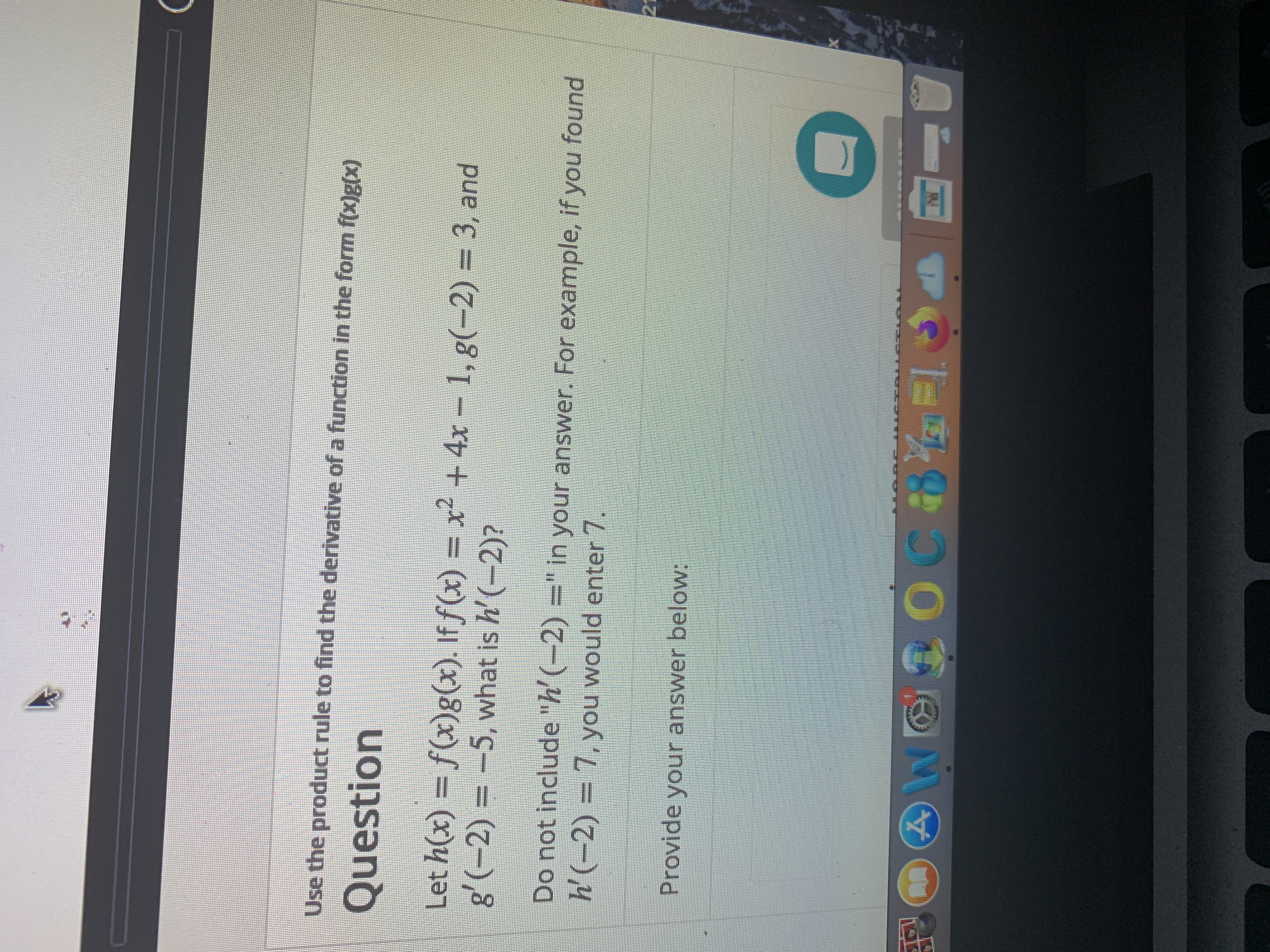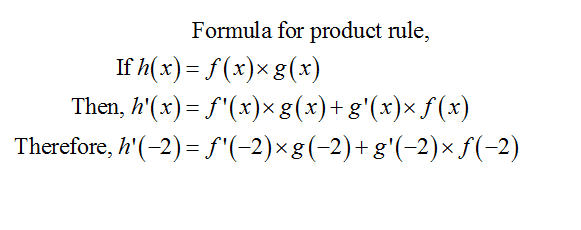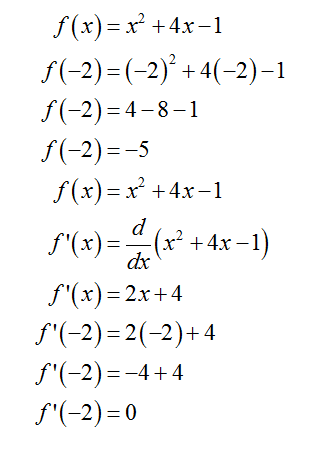# Use the product rule to find the derivative of a function in the form f(x]g(x)QuestionLet h(x) = f(x)g(x). If f(x) = x² +4xg'(-2) = -5, what is h' (-2)?1, g(-2) = 3, andDo not include "h' (-2) =" in your answer. For example, if you foundh'(-2) = 7, you would enter 721Provide your answer below:

Question
1 viewshelp_outlineImage TranscriptioncloseUse the product rule to find the derivative of a function in the form f(x]g(x) Question Let h(x) = f(x)g(x). If f(x) = x² +4x g'(-2) = -5, what is h' (-2)? 1, g(-2) = 3, and Do not include "h' (-2) =" in your answer. For example, if you found h'(-2) = 7, you would enter 7 21 Provide your answer below: fullscreen
check_circle

star
star
star
star
star
1 Rating
Step 1

First of all, consider the formula for the product rule to calculate the derivative of the function in the form of f(x)g(x).

Substitute the value of the x = -2 in the derivative of h(x).Step 2

Then, substitute the value of x = -2 in the f (x) and calculate f (-2).

Now, differentiate f (x) w.r.t x and find the derivative of f (x).

Again, Substitute the value of x = -2 in the derivative of f (x) and calculate the value of the derivative at x = -2....

### Want to see the full answer?

See Solution

#### Want to see this answer and more?

Solutions are written by subject experts who are available 24/7. Questions are typically answered within 1 hour.*

See Solution
*Response times may vary by subject and question.
Tagged in

### Calculus